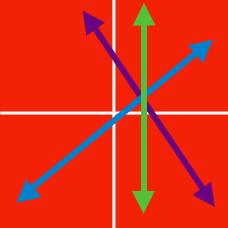Algebra

# System of Linear Equations: Level 3 Challenges

$\large{ \begin{cases} 5a+3b+c=17 \\ 1a + 4b+7c=21 \end{cases}}$

Given that $a,b$ and $c$ satisfy the system of equations above, and $a+b+c$ is equal to $\dfrac xy$, where $x$ and $y$ are coprime positive integers, find $x+y$.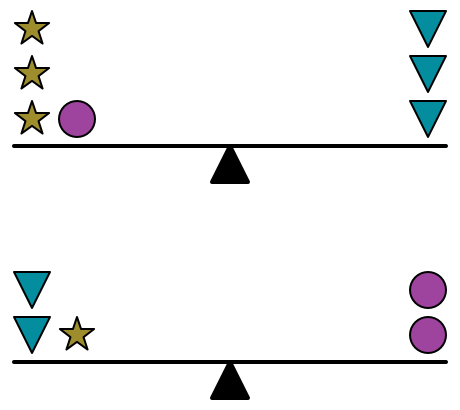In the diagram to the right, both beams are balanced.

Which of the following options will not balance any of the other options?

Note that there are 5 options (not 4).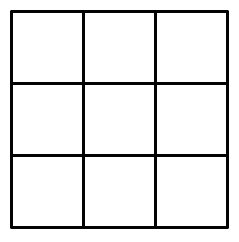I filled up a $3 \times 3$ grid with numbers, such that if we pick any 2 (not necessarily consecutive) rows and columns, the sum of the 4 numbers in their intersection is equal to 0.

Which is the most specific/restrictive sentence that we say about the numbers in the grid?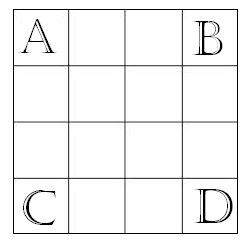A magic square of order 4 is created by putting the integers 1 to 16 into a 4 by 4 square grid so that the sum of the numbers in each row, column and main diagonal is the same. What is the sum of the integers at the corners of this magic square (labeled by letters as shown above)?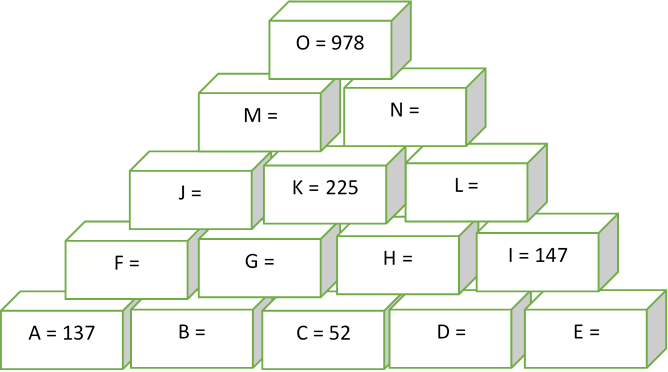Every brick in this pyramid contains a number that is the sum of the two numbers in the two bricks below it. What is the value of $B$?

×